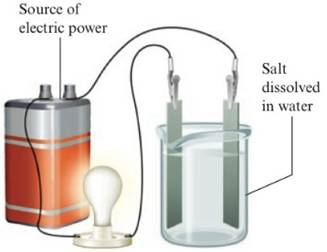Chapter 4, Problem 79QAPIntroductory Chemistry: A Foundati...

9th Edition
Steven S. Zumdahl + 1 other
ISBN: 9781337399425

Solutions

Chapter
SectionIntroductory Chemistry: A Foundati...

9th Edition
Steven S. Zumdahl + 1 other
ISBN: 9781337399425
Textbook Problem

List some properties of a substance that would lead you to believe it consists of ions. How do these properties differ from those of nonionic compounds?Interpretation Introduction

Interpretation:

The properties of ionic and non-ionic compounds should be explained.

Concept Introduction:

The compounds which can form ions in their solution are called as ionic compounds whereas non-ionic compounds cannot give ions in their solutions.

Explanation

Ionic compounds are mainly formed by ions. Here ions are bonded through strong electrostatic force of attraction. Hence some common properties of compounds that reflects the prese...

Still sussing out bartleby?

Check out a sample textbook solution.

See a sample solution

The Solution to Your Study Problems

Bartleby provides explanations to thousands of textbook problems written by our experts, many with advanced degrees!

Get Started

Find more solutions based on key concepts